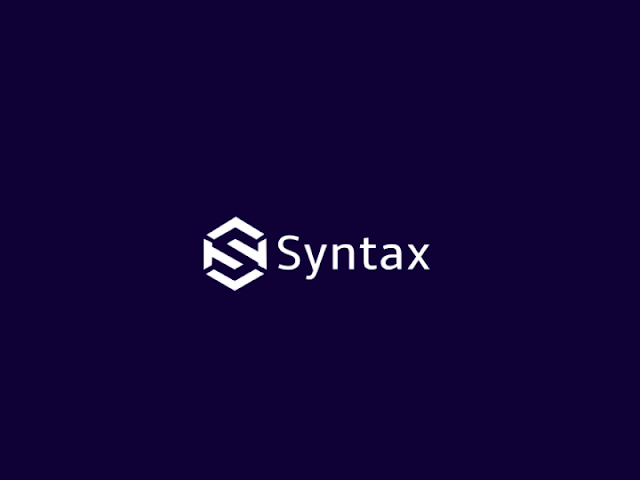• For 3-D rotation, we need to pick an axis to rotate an object.

• The most common choices are the x-axis, y-axis, and z-axis.

# X-Axis Rotation

• The transformation for x-axis is obtain from equation of z-axis rotation by replacing cyclically as shown here:

x -> y -> z -> x

• Rotation about x-axis, we leave x co-ordinate unchanged.

y' = ycosθ - zsinθ

z' = ysinθ + zcosθ

x' = x

• Matrix equation is:

p' = Rx (θ) * p

# Y-Axis Rotation

The transformation for y-axis is obtain from equation of x-axis rotation by replacing cyclically as shown here:

x -> y -> z -> x

• Rotation about y-axis, we leave y co-ordinate unchanged.

z' = zcosθ - xsinθ

x' = zsinθ + xcosθ

y' = y

• Matrix equation is:

p' = Ry (θ) * p

# Z-Axis Rotation

• Two-dimension rotation equation can be easily converted into 3-D z-axis rotation equation.

• Rotation about z-axis, we leave z co-ordinate unchanged.

x' = xcosθ - ysinθ

y' = xsinθ + ycosθ

z' = z

• Where, parameter θ specify rotation angle.

• Matrix equation is:

p' = Rz (θ) * p

String Handling In Java

Comment your views on this Article :)

## Thank you for visiting my blog :)

#### No comments

Comment your views on this article

### Syntax Explanation of System Software Programs